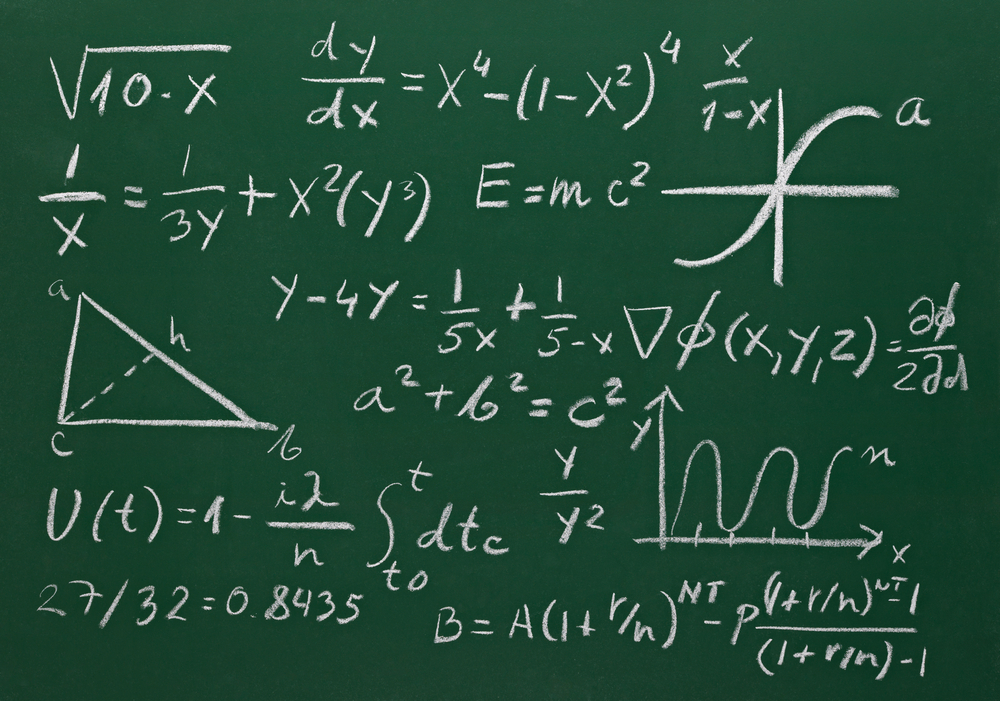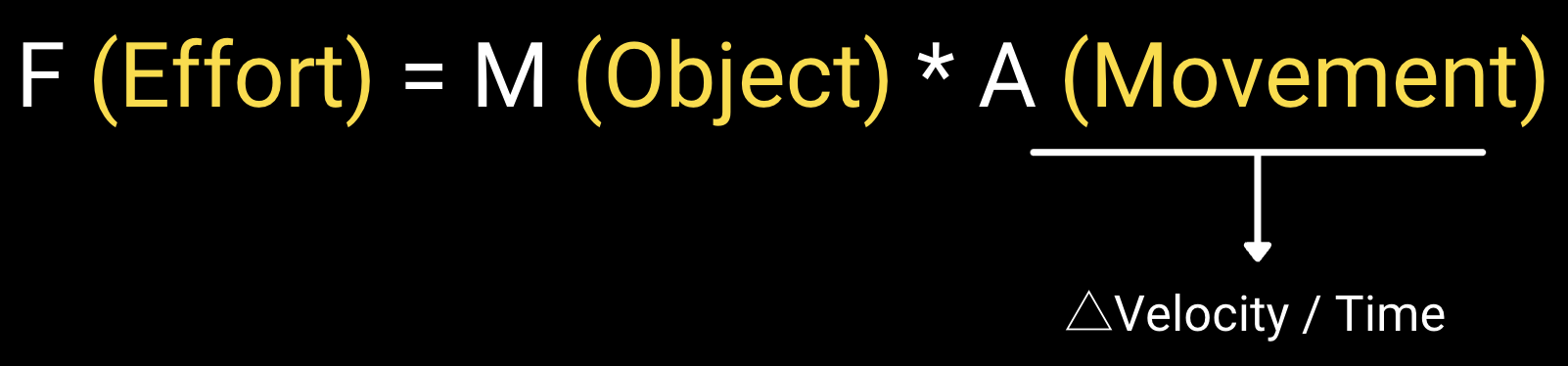Force Plate

# From force to velocity: What is this wizardry?

Introduction So, we now know that we can get an awful lot of fascinating and useful information from simple tests ...

# Introduction

So, we now know that we can get an awful lot of fascinating and useful information from simple tests performed on a force plate measurement system.

We also know that force is how hard you push or pull something.How then, can we go from knowing how hard something is pushed or pulled to knowing how fast it moves? Well, this is an important question because being able to calculate how fast something moves lets us calculate the following...

• How far it moves
• The direction it moves in
• The work performed and the power achieved by moving it

Now, if you've learned anything from my blog posts it's the power of understanding where your data comes from and it's because of the three points I just listed that I think it's important that you understand how we get velocity from force.

# Wizardry or good, old fashioned maths?

Of course, I appreciate that maths (yes, I'm English - we invented the language and that's what we call it) isn't necessarily everyone's cup of tea, but don't fret, I'm no mathematician so I'll do my best to take the worst of the sting out of the following explanation.So, hopefully we all know that if you know how far something's moved and you know how long it took to move that we can calculate its speed? Essentially, we take how far it's moved and divide it by how long it took to move. If we're not worried about the direction the object of interest has moved in then we can refer to the result as speed. However, if we are interested in the direction the object of interest has moved in -- and let's face it, direction is a pretty important part of sport -- then we'd refer to it as velocity.

We could repeat this process, dividing velocity by time to calculate acceleration -- whether the object of interest is speeding up, slowing down, or moving at a constant velocity. We've all come across the equation that is often used to describe Newton's second law of motion...

F = ma...that's right: force = mass × acceleration. If we know the acceleration of the object of interest, and we know it's mass we can multiply these to get force. Technically, this gives us what we often refer to as net force, the force that influences movement. If we think back to the bathroom scale analogy of a force plate that I gave a few blog articles ago we can think of net force as force minus body weight. This, remember, is because just by standing still we apply force to the ground. However, that doesn’t move us. It’s the force that’s either greater or less than our body weight that does this.

# Reversing this: Numeric Integration

Numerical differentiation is the long-winded way of saying we’ve divided something (say, how far something moves) by time (how long it takes to move).

The opposite of numeric differentiation is numeric integration.. simple, right?

That’s right, instead of dividing some metrics by time to calculate other metrics, we multiply them by time.

If we know how much force has been applied to a known mass (our jumper – and we do, that’s why we’re using the force plate in the first place, right?), then we can calculate the following:

• Net force = force minus body weight
• Acceleration = net force ÷ body mass (body weight ÷ the acceleration of gravity [9.81 m/s/s])
• Velocity = acceleration × time
• Displacement = velocity × timeAnd this is why force plate companies should be open with their customers and operate under full transparencies. Remember to ask yourself this really important question:

• How can I take ownership of my data if I don’t know where it’s come from?

Of course, it isn’t always as simple as this. For example, in the above example we’re calculating the average velocity. Typically, force plate software will calculate metrics like net force, acceleration, velocity and displacement on a sample-by-sample basis. This actually doesn’t add any complexity to the data processing, it just means that your data will be integrated one row at a time. Of course, once we have velocity we can multiply it by force to get power (often a fluffy, unnecessary metric, but it definitely has its place). Interestingly, if we’re interested in how much work has been performed by pushing the object of interest to move then we can integrate phase average power with respect to time (e.g. propulsion work = propulsion phase average power × time).# Simple, right?

Well, yes. It really is. There really isn’t any wizardry involved in force data processing. As a consequence, there really shouldn’t be any secrecy either. However, we must end this on a cautionary note…

The calculations that any force measurement system software will do on your data will only be as good as the force data you give it. What I mean by this is that you must ensure that you standardize (see the last blog article) any tasks that your athletes or clients do on your force plates. The further you let your athletes or clients get away from any standardized task, the less accurate your data will be and the less confidence you’ll be able to have in them. So remember…

• Provide your athletes and clients with clear instructions
• Give your athletes and clients sufficient time to practice the task(s) you want them to do
• Follow a script of instructions to issue for each trial
• Watch your athletes or clients perform each trial (and repeat it if you’re not happy with it

I hope this article has helped to shed a bit of light onto an element of the force plate system that often seems to be shrouded in mystery. Remember, if anyone tries to tell you that it’s more complicated than this then they’re probably either trying to sell you something or they have something to hide. Probably both.

Finally, if you have any questions then please drop me an email at Jason@HawkinDynamics.com

>> Now that you've learned about force & velocity, read the CMJ Playbook to learn how it applies to Human Movement.